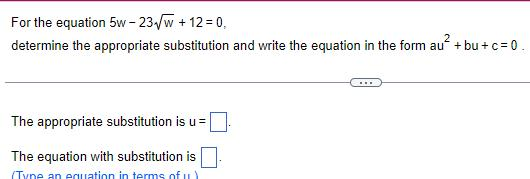Question:

# For the equation 5w 23 w 12 0 determine the appropriate

Last updated: 9/20/2023For the equation 5w 23 w 12 0 determine the appropriate substitution and write the equation in the form au bu c 0 The appropriate substitution is u The equation with substitution is Tyne an equation in terms of u